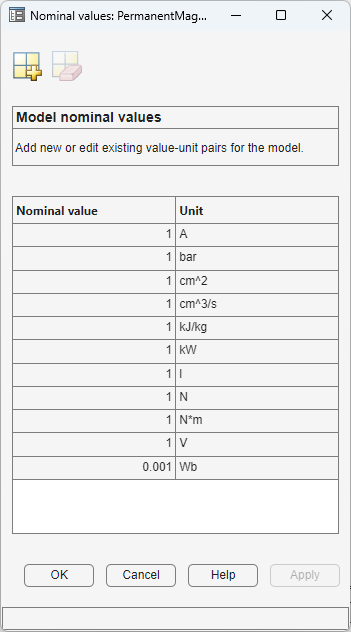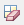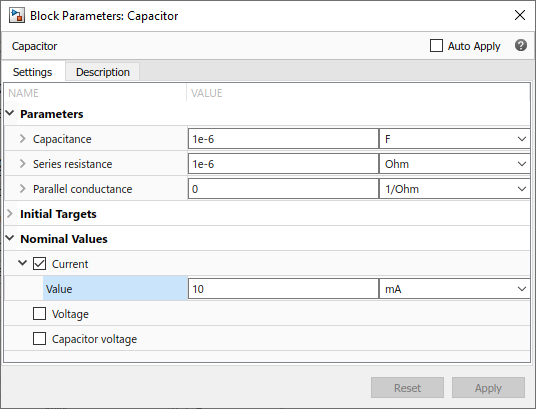## System Scaling by Nominal Values

Nominal values provide a way to specify the expected magnitude of a variable in a model, similar to specifying a transformer rating, or setting a range on a voltmeter. Using system scaling based on nominal values increases the simulation robustness. This functionality provides a way to fine-tune scaling of individual variables in a model. It is especially helpful for initial conditions convergence and maintaining a minimum step size.

### Enable or Disable System Scaling by Nominal Values

Using system scaling based on nominal values is a best practice for Simscape™ models because it improves simulation robustness. Therefore, when you create a new model, scaling by nominal values is enabled by default.

System scaling by nominal values is controlled by the Normalize using nominal values configuration parameter.

1. In the Simulink® Toolstrip at the top of the model window, open the Modeling tab and click Model Settings. The Configuration Parameters dialog box opens.

2. In the Configuration Parameters dialog box, in the left pane, select Simscape. The right pane displays the Normalize using nominal values check box:

• If the check box is selected, the model provides the scaling information to the solver based on the specified nominal values. To view, add, and edit the value-unit pairs for the model, click the Specify nominal values button next to the Normalize using nominal values check box.

• If the check box is cleared, the scaling by nominal values is disabled.

### Possible Sources of Nominal Values and Their Evaluation Order

The scaling of each variable is determined by its nominal value and physical units. Nominal values can come from different sources:

• Block — You can specify nominal value and unit as variable declaration attributes in a Simscape component file underlying the block. These attributes translate into block parameters `x_nominal_value` and `x_nominal_unit` (where `x` is the variable name). You can also override these values on individual blocks in the model by setting the corresponding block parameter `x_nominal_specify` to `'on'` and supplying different values for `x_nominal_value` and `x_nominal_unit`. You can use the block dialog box, the Property Inspector, or `set_param` and `get_param` functions to view and change the values of these parameters. For more information, see Modify Nominal Values for a Block Variable.

• Model — In absence of a nominal value specified for the block, a variable uses the nominal value for the commensurate physical unit specified in the model table. All models have a default table of nominal values and units (factory default). To view, add, and edit the value-unit pairs for the model, click the Specify nominal values button next to the Normalize using nominal values check box. For more information, see Specify Nominal Value-Unit Pairs for a Model.

• Derived — If the model table of nominal values does not contain a row for a unit commensurate with the physical unit of a variable, then the nominal value for this variable is derived from fundamental dimensions. For example, if the variable's initial value is in `lbf`, and there is no entry in the table for force, but the table contains `{10,'lbm'}`, `{12,'ft'}`, and `{2,'min'}`, then the nominal value for that variable is `{10*12/2^2,'lbm*ft/min^2'}`.

• Fixed — Event variables, top-level model inputs, and Simscape Multibody™ variables cannot be scaled according to nominal values.

The Variable Viewer in advanced configuration shows the nominal value and unit for each variable, along with the source. For more information, see Variable Viewer.

### Specify Nominal Value-Unit Pairs for a Model

All models have a default table of nominal values and units (factory default). To view, add, and edit the value-unit pairs for a model:

1. In the Simulink Toolstrip at the top of the model window, open the Modeling tab and click Model Settings. The Configuration Parameters dialog box opens.

2. In the Configuration Parameters dialog box, in the left pane, select Simscape.

3. Make sure the Normalize using nominal values check box is selected.

4. Click the Specify nominal values button next to the Normalize using nominal values check box.

The model table of nominal values opens in a new window. It contains all the value-unit pairs currently defined for the model.5. To edit a value-unit pair, select the corresponding cell and enter the new value or unit.

6. To add a new value-unit pair, in the top toolbar of the window containing the table, click. This action adds a new empty row at the bottom of the table. Select the cells in this row and enter the nominal value and unit for the new value-unit pair.

7. To delete a value-unit pair, select the corresponding row and click.

8. When finished editing the table, click . Table data is saved when you save the model.

### Modify Nominal Values for a Block Variable

Each variable in a block has three associated block parameters (where `x` is the variable name):

• `x_nominal_specify` — Lets you override the system default nominal value for variable `x` in this particular block. The default parameter value is `'off'`, in which case the variable nominal value is determined according to the evaluation order described in Possible Sources of Nominal Values and Their Evaluation Order. Set this parameter to `'on'` to use the `x_nominal_value` and `x_nominal_unit` parameter values for scaling.

• `x_nominal_value` — If the `x_nominal_specify` parameter is set to `'on'`, then this value, in conjunction with the nominal unit parameter, determines the scaling of variable `x` in this particular block. The parameter value must be a numeric value, specified as a character vector. The default parameter value is `'1'`.

• `x_nominal_unit` — If the `x_nominal_specify` parameter is set to `'on'`, then this unit, in conjunction with the nominal value parameter, determines the scaling of variable `x` in this particular block. The parameter value must be a valid physical unit name, specified as a character vector. The unit must be commensurate with the unit specified for the initial value of the variable. The default unit is the same as for the initial value.

Note

Nominal value and unit can be specified as variable declaration attributes in a Simscape component file underlying the block. For more information, see Nominal Value and Unit for a Variable. In this case, the nominal value and unit parameters for that variable get their default values from the variable declaration attributes.

You can use `set_param` and `get_param` functions to view and change the values of these parameters.

For example, to change the nominal value and unit for variable `i` (current) for an individual block, select this block in the model and type:

```set_param(gcb,'i_nominal_specify','on') set_param(gcb,'i_nominal_value','10') set_param(gcb,'i_nominal_unit','mA')```

This sequence of commands overrides the default nominal value for the block variable and sets it to 10 mA.

To perform the same actions using the block dialog box:

1. Double-click the block in the model.

2. In the block dialog box, expand the Nominal Values section.3. Select the check box next to Current. This action is equivalent to setting the `i_nominal_specify` parameter to `'on'`.

4. Once the Current check box is selected, its Value field becomes editable. Enter `10` and select `mA` from the unit drop-down list.